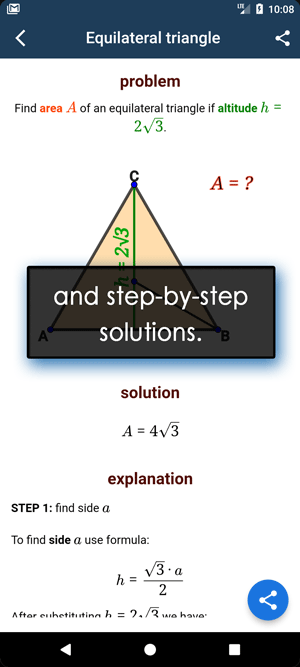Math Calculators, Lessons and Formulas

It is time to solve your math problem

mathportal.org

# Prime factorization calculator

Calculator finds prime factorization of a given number and creates a factor tree. The calculator generates a step by step explanation on how the work has been done.

Prime Factorization Calculator
that shows a step by step explanation
show help ↓↓ examples ↓↓
Find prime factorization and factor tree
Find all factors
Find all factor pairs
Check if the number is prime or notworking...
examples
example 1:ex 1:
Create factor tree for 154 ?
example 2:ex 2:
Find prime factorization of 70 and create factor tree?
example 3:ex 3:
What are the prime factors of 18240 ?
example 4:ex 4:
Find all factor pairs of 548.
example 5:ex 5:
Is the number 1361 prime or composite?

### Finding prime factorization and factor tree

Example: Find prime factorization of 60.

Step 1: Start with any number that divides 60, in this we will use 10. So, $\color{blue}{60 = 6 \cdot 10}$.

• 60
• 10
• 6

Step 2: Write 10 as a product of 2 and 5. $\color{blue}{10 = 2 \cdot 5}$.

• 60
• 10
• 5
• 2
• 6

Step 3: Write 6 as a product of 2 and 3. $6 = 2 \cdot 3$.

• 60
• 10
• 5
• 2
• 6
• 2
• 3

Step 4: The end nodes are the prime factors of 60.

• 60
• 10
• 5
• 2
• 6
• 2
• 3
$$60 = 2 \cdot 2 \cdot 3 \cdot 5$$

### Finding all factors

Example: Find all factors of 54.

Step 1: Begin the list with 1 and end it with 54.

$$\color{blue}{\boxed{1}} ~ , ~ . ~ . ~ . ~ , ~ \color{blue}{\boxed{54}}$$

Step 2: Since $54 = 2 \cdot 27$ we put in 2 and 27 into an array.

$$1 ~ , ~ \color{blue}{\boxed{2}} ~ , ~ . ~ . ~ . ~ , ~ \color{blue}{\boxed{27}} ~ , ~ 54$$

Step 3: Since $54 = 3 \cdot 18$ so we will put in 3 and 18 into an array.

$$1 ~ , ~ 2, ~ \color{blue}{\boxed{3}} ~ , ~ . ~ . ~ . ~ , ~ \color{blue}{\boxed{18}} ~ , ~ 27 ~ , ~ 54$$

Step 4:At the end we have $54 = 6 \cdot 9$

$$1 ~ , ~ 2 ~ , ~ 3 ~ , ~ \color{blue}{\boxed{6}} ~ , ~ \color{blue}{\boxed{9}} ~ , ~ 18 ~ , ~ 27 ~ , ~ 54$$

### Check if the number is prime

Example: Check whether a number 581 is prime or not.

Step 1: Find the square root of 581.

$$\sqrt{581} = 24.1 \approx = 25$$

Step 2: Try to divide 581 by all prime numbers less or equal to 25.

In this example we will try to divide 581 with :
2 , 3, 5, 7, 11, 13, 17, 19 and 23.

\begin{aligned} 581 : 2 & = 290.5 \\ 581 : 3 & = 484 \\ 581 : 5 &= 116.2 \\ 581 : 7 &= 83 \end{aligned}

581 is divisible by 3 so is is not prime.

Search our database of more than 200 calculators